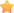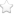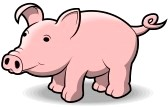×• A 比 D 多買 23 隻豬。
• B 比 E 少買 19 隻豬。
• 每個人所買到的豬隻數目，剛好等於買到豬隻的單價錢數。
• 每位丈夫都比他的妻子多花 63 元買豬。◎本題引用自遊戲學校，網友mightqxc提供

A 的妻子是 E；
B 的妻子是 D；
C 的妻子是 F。

63 = 1 × 63 = 3 × 21 = 7 × 9 =  (H-W)(H+W)     共 3 種

H = 32, W = 31 ； H = 12, W = 9 ； H = 8, W = 1

(至於他們買幾隻豬不能確定，只知道也是以上三組解的其中之一)。

（你有更好的解法嗎？歡迎到粉絲團遊戲學校去跟大家分享喔！）